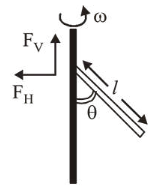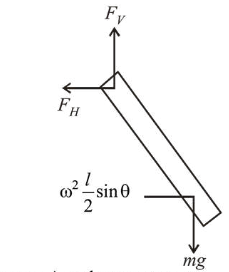# A uniform rod of lengthQuestion:A uniform rod of length ' $l$ ' is pivoted at one of its ends on a vertical shaft of negligible radius. When the shaft rotates at angular speed $\omega$ the rod makes an angle $\theta$ with it (see figure). To find $\theta$ equate the rate of change of angular momentum (direction going into the paper)

$\frac{m l^{2}}{12} \omega^{2} \sin \theta \cos \theta$ about the centre of mass (CM) to the\

torque provided by the horizontal and vertical forces $F_{H}$ and $F_{V}$ about the CM. The value of $\theta$ is then such that:

1. $\cos \theta=\frac{2 g}{3 l \omega^{2}}$

2. $\cos \theta=\frac{g}{2 l \omega^{2}}$

3. $\cos \theta=\frac{g}{l \omega^{2}}$

4. $\cos \theta=\frac{3 g}{2 l \omega^{2}}$

Correct Option: 4,

Solution:

(4) Vertical force $=m g$

Horizontal force $=$ Centripetal force $=m \omega^{2} \frac{l}{2} \sin \theta$

Torque due to vertical force $=m g \frac{l}{2} \sin \theta$

Torque due to horizontal force $=m \omega^{2} \frac{l}{2} \sin \theta \frac{l}{2} \cos \theta$Net Torque $=$ Angular momentum

$m g \frac{l}{2} \sin \theta-m \omega^{2} \frac{l}{2} \sin \theta \frac{l}{2} \cos \theta=\frac{m l^{2}}{12} \omega^{2} \sin \theta \cos \theta$

$\Rightarrow \cos \theta=\frac{3}{2} \frac{g}{\omega^{2} l}$